• 使用matlab的分水岭算法对骨关节图像进行分割，基于梯度下降的方法进行优化
• 基于组平均距离聚类算法 层次聚类算法 cluster group average-distance
•研究论文
• 提出一种基于平均海明距离的无线传感器网络安全路由算法。该算法利用节点的实时包传递率短序列和正常运行短序列之间的平均海明距离，检测节点是否正常。在节点中引入邻近节点表，对恶意节点进行隔离，在重新组簇的...
• 为了提高DV-Hop定位算法在不规则网络中的精确度，针对该算法的不足之处，提出了一种基于平均跳距和估计距离的改进的DV-Hop算法。该算法考虑了未知节点到参考节点的路径与参考节点间的路径可能存在重合或部分重合这一...无线传感器网络
• 基于层次的聚类方法 简介 基于层次的聚类方法 概念 聚合层次聚类 图示 ...族间距离 平均距离 基于层次聚类 ( 聚合层次聚类 ) 步骤 基于层次聚类 ( 聚合层次聚类 ) 算法终止条件 族半径 计算公式 基于层次聚类总结


文章目录
基于层次的聚类方法 简介基于层次的聚类方法 概念聚合层次聚类 图示划分层次聚类 图示基于层次的聚类方法 切割点选取族间距离 概念族间距离 使用到的变量族间距离 最小距离族间距离 最大距离族间距离 中心点距离族间距离 平均距离基于层次聚类 ( 聚合层次聚类 ) 步骤基于层次聚类 ( 聚合层次聚类 ) 算法终止条件族半径 计算公式基于层次聚类总结

基于层次的聚类方法 简介

1 . 基于层次的聚类方法 : 将 数据集样本对象 排列成 聚类树 , 在 指定 的层次 ( 切割点 ) 进行切割 , 切割点 时刻 的聚类分组 , 就是 最终需要的聚类分组 ; 也就是这个切割点的切割的时刻 , 互相关联的样本 , 划分到一个聚类分组中 ;

2 . 基于层次聚类方法 的两种方式 :

① 聚合层次聚类 : 开始时 , 每个对象都是一个聚类分组 ( 原子聚类 ) , 根据 聚类之间的相似性 , 对原子聚类逐渐合并 , 最终会合并成一个聚类 ; 其 本质是 由 多个聚类分组 切割成 成少数 聚类分组 ;

② 划分层次聚类 : 开始时 , 所有的样本都在一个聚类中 , 根据聚类间相似性 , 对聚类进行划分 , 最终 每个样本 都会被划分成一个聚类分组 ( 原子聚类 ) ; 本质是 由 少数 聚类分组 划分成多个 聚类分组 ;

基于层次的聚类方法 概念

1 . 基于层次的聚类方法 概念 : 将数 据集样本对象 排列成 树结构 , 称为 聚类树 , 在指定的层次 ( 步骤 ) 上切割数据集样本 , 切割后时刻的 聚类分组 就是 聚类算法的 聚类结果 ;

2 . 基于层次的聚类方法 : 一棵树可以从叶子节点到根节点 , 也可以从根节点到叶子节点 , 基于这两种顺序 , 衍生出两种方法分支 , 分别是 : 聚合层次聚类 , 划分层次聚类 ;

3 . 聚合层次聚类 ( 叶子节点到根节点 ) : 开始时 , 每个样本对象自己就是一个聚类 , 称为 原子聚类 , 然后根据这些样本之间的 相似性 , 将这些样本对象 ( 原子聚类 ) 进行 合并 ;
常用的聚类算法 : 大多数的基于层次聚类的方法 , 都是 聚合层次聚类 类型的 ; 这些方法从叶子节点到根节点 , 逐步合并的原理相同 ; 区别只是聚类间的相似性计算方式不同 ;

4 . 划分层次聚类 ( 根节点到叶子节点 ) : 开始时 , 整个数据集的样本在一个总的聚类中 , 然后根据样本之间的相似性 , 不停的切割 , 直到完成要求的聚类操作 ;

5 . 算法性能 : 基于层次的聚类方法的时间复杂度为

O

(

N

2

)

O(N^2)

, 如果处理的样本数量较大 , 性能存在瓶颈 ;

聚合层次聚类 图示

1 . 聚合层次聚类 图示 :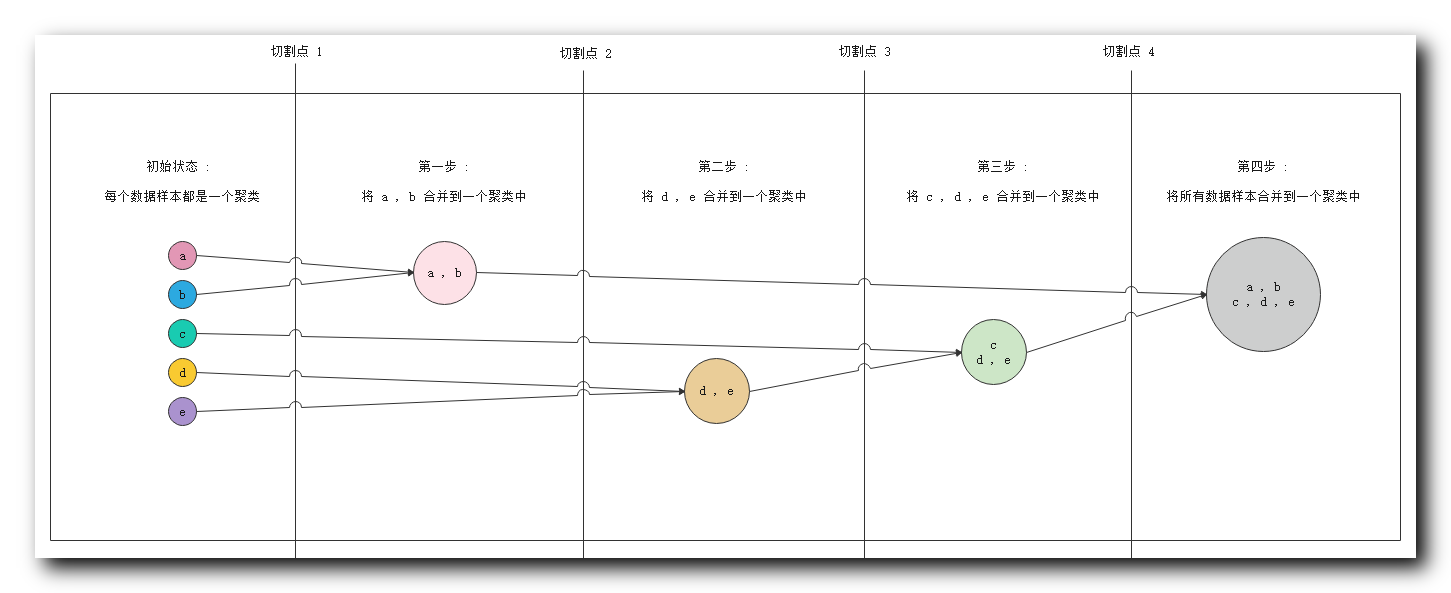① 初始状态 : 最左侧 五个 数据对象 , 每个都是一个聚类 ;
② 第一步 : 分析相似度 , 发现

a

,

b

a , b

相似度很高 , 将

{

a

,

b

}

\{a ,b\}

分到一个聚类中 ;
③ 第二步 : 分析相似度 , 发现

d

,

e

d, e

相似度很高 , 将

{

d

,

e

}

\{d, e\}

分到一个聚类中 ;
④ 第三步 : 分析相似度 , 发现

c

c

与

d

,

e

d,e

相似度很高 , 将

c

c

数据放入

{

d

,

e

}

\{d, e\}

聚类中 , 组成

{

c

,

d

,

e

}

\{c,d, e\}

聚类 ;
⑤ 第四步 : 分析相似度 , 此时要求的相似度很低就可以将不同的样本进行聚类 , 将前几步生成的两个聚类 , 合并成一个聚类

{

a

,

b

,

c

,

d

,

e

}

\{a, b, c, d, e\}

;

2 . 切割点说明 : 实际进行聚类分析时 , 不会将所有的步骤走完 , 这里提供四个切割点 , 聚类算法进行聚类时 , 可以在任何一个切割点停止 , 使用当前的聚类分组当做聚类结果 ;

① 切割点

1

1

: 在切割点

1

1

停止 , 会得到

5

5

个聚类分组 ,

{

a

}

\{a\}

,

{

b

}

\{b\}

,

{

c

}

\{c\}

,

{

d

}

\{d\}

,

{

e

}

\{e\}

;
② 切割点

2

2

: 在切割点

2

2

停止 , 会得到

4

4

个聚类分组 ,

{

a

,

b

}

\{a, b\}

,

{

c

}

\{c\}

,

{

d

}

\{d\}

,

{

e

}

\{e\}

;
③ 切割点

3

3

: 在切割点

3

3

停止 , 会得到

3

3

个聚类分组 ,

{

a

,

b

}

\{a, b\}

,

{

c

}

\{c\}

,

{

d

,

e

}

\{d, e\}

;
④ 切割点

4

4

: 在切割点

4

4

停止 , 会得到

2

2

个聚类分组 ;

{

a

,

b

}

\{a, b\}

,

{

c

,

d

,

e

}

\{c, d, e\}

;
⑤ 走完整个流程 : 会得到

1

1

个聚类分组 ,

{

a

,

b

,

c

,

d

,

e

}

\{a, b ,c, d, e\}

;

划分层次聚类 图示

1 . 划分层次聚类 图示 :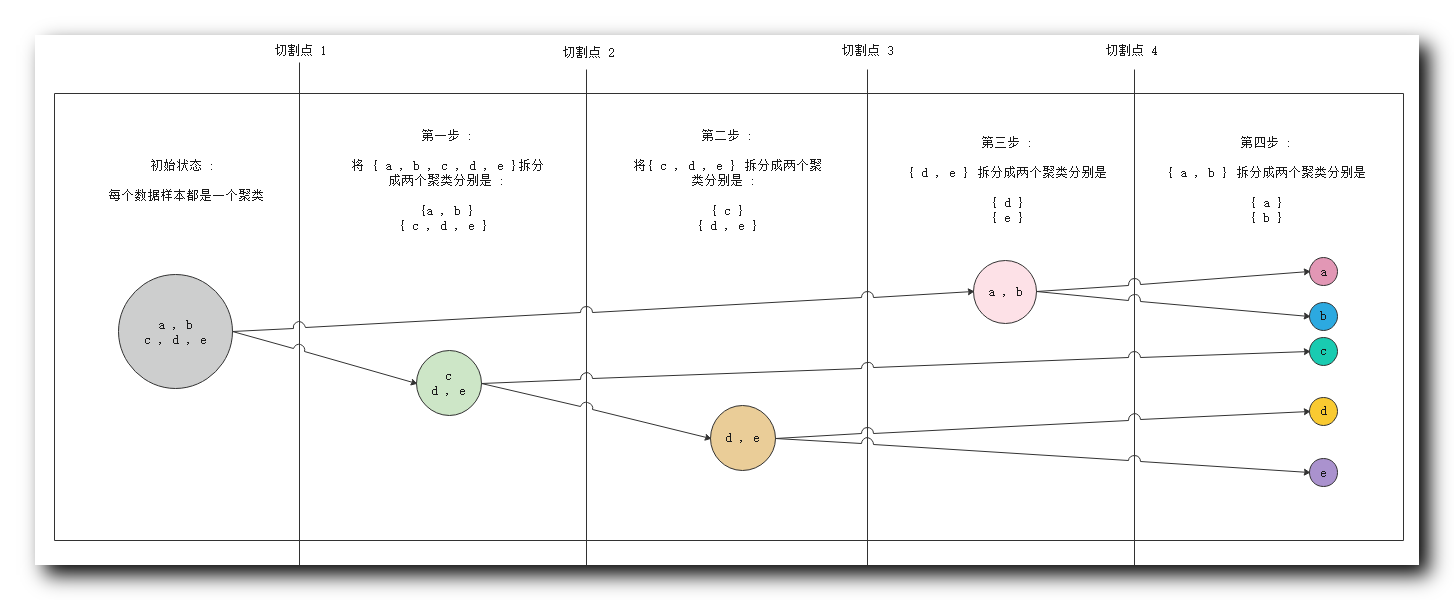① 初始状态 : 最左侧 五个 数据对象 , 属于一个聚类 ;
② 第一步 : 分析相似度 , 切割聚类 , 将

{

c

,

d

,

e

}

\{c,d, e\}

与

{

a

,

b

}

\{a ,b\}

划分成两个聚类 ;
③ 第二步 : 分析相似度 , 将

{

c

,

d

,

e

}

\{c,d, e\}

中的

{

c

}

\{c\}

与

{

d

,

e

}

\{d, e\}

划分成两个聚类 ;
④ 第三步 : 分析相似度 , 将

{

d

,

e

}

\{d, e\}

拆分成

{

d

}

\{d\}

和

{

e

}

\{e\}

两个聚类 ;
⑤ 第四步 : 分析相似度 , 将

{

a

,

b

}

\{a ,b\}

拆分成

{

a

}

\{a\}

和

{

b

}

\{b\}

两个聚类 , 至此所有的数据对象都划分成了单独的聚类 ;

2 . 切割点说明 : 实际进行聚类分析时 , 不会将所有的步骤走完 , 这里提供四个切割点 , 聚类算法进行聚类时 , 可以在任何一个切割点停止 , 使用当前的聚类分组当做聚类结果 ;

① 切割点

1

1

: 在切割点

1

1

停止 , 会得到

1

1

个聚类分组 ,

{

a

,

b

,

c

,

d

,

e

}

\{a, b ,c, d, e\}

;
② 切割点

2

2

: 在切割点

2

2

停止 , 会得到

2

2

个聚类分组 ;

{

a

,

b

}

\{a, b\}

,

{

c

,

d

,

e

}

\{c, d, e\}

;
③ 切割点

3

3

: 在切割点

3

3

停止 , 会得到

3

3

个聚类分组 ,

{

a

,

b

}

\{a, b\}

,

{

c

}

\{c\}

,

{

d

,

e

}

\{d, e\}

\$ ;
④ 切割点

4

4

: 在切割点

4

4

停止 , 会得到

4

4

个聚类分组 ,

{

a

,

b

}

\{a, b\}

,

{

c

}

\{c\}

,

{

d

}

\{d\}

,

{

e

}

\{e\}

;
⑤ 走完整个流程 : 会得到

5

5

个聚类分组 ,

{

a

}

\{a\}

,

{

b

}

\{b\}

,

{

c

}

\{c\}

,

{

d

}

\{d\}

,

{

e

}

\{e\}

;

基于层次的聚类方法 切割点选取

1 . 算法终止条件 ( 切割点 ) : 用户可以指定聚类操作的算法终止条件 , 即上面图示中的切割点 , 如 :

① 聚类的最低个数 : 聚合层次聚类中 ,

n

n

个样本 , 开始有

n

n

个聚类 , 逐步合并 , 聚类个数逐渐减少 , 当聚类个数达到最低值

m

i

n

min

, 停止聚类算法 ;
② 聚类最高个数 : 划分层次聚类中 ,

n

n

个样本 , 开始有

1

1

个聚类 , 逐步划分 , 聚类个数逐渐增加 , 当聚类个数达到最大值

m

a

x

max

, 停止聚类算法 ;
③ 聚类样本的最低半径 : 聚类的数据样本范围不能无限扩大 , 指定一个阈值 , 只有将该阈值内的样本放入一组 ; 半径指的是所有对象距离其平均点的距离 ;

2 . 切割点回退问题 : 切割点一旦确定 , 便无法回退 ; 这里以聚合层次聚类为例 :

① 处于切割点

4

4

: 如已经执行到了步骤三 , 此时处于切割点

4

4

, 聚类分组为

{

a

,

b

}

\{a, b\}

,

{

c

,

d

,

e

}

\{c, d, e\}

;
② 试图回退到 切割点

3

3

: 想要会回退到切割点

3

3

的状态 , 视图将聚类分组恢复成

{

a

,

b

}

\{a, b\}

,

{

c

}

\{c\}

,

{

d

,

e

}

\{d, e\}

;
③ 无法回退 : 该操作是无法实现的 , 聚类分组一旦 合并 或 分裂 , 此时就无法回退 ;

族间距离 概念

族间距离 :

① 作用: 族间距离 , 就是聚类分组之间的距离 , 之前的距离计算都是 样本 之间的距离 , 这里的基于层次聚类时 , 不管是聚合层次聚类 , 还是划分层次聚类 , 其都要进行 聚类分组 间的相似度比较 ,
② 聚合层次聚类 : 是 根据 聚类的族间距离 ( 聚类分组相似性 ) 将不同的聚类分组进行合并 ;
③ 划分层次聚类 : 是 根据 聚类的族间距离 ( 聚类分组相似性 ) 将不同的聚类分组进行划分 ( 拆分 ) ;

族间距离 使用到的变量

公式中 用到的 变量 :

① 样本表示 :

p

p

和

q

q

表示 分别 处于两个聚类分组中的 两个样本 ;
② 样本距离表示 :

d

(

p

,

q

)

d(p, q)

表示

p

p

样本对象 与

q

q

样本对象的距离 ;
③ 聚类 ( 族 ) 表示 :

C

i

C_i

和

C

j

C_j

分别表示两个 聚类 / 族 / 聚类分组 ;
④ 聚类距离表示 :

d

(

C

i

,

C

j

)

d(C_i, C_j)

表示

C

i

C_i

聚类 与

C

j

C_j

聚类 之间的距离 ;
⑤ 聚类中心点 :

m

i

m_i

是

C

i

C_i

聚类的中心点 ,

m

j

m_j

是

C

j

C_j

聚类的中心点 ;
⑥ 样本个数 :

n

i

n_i

是

C

i

C_i

聚类的样本个数 ,

n

j

n_j

是

C

j

C_j

聚类的样本个数 ;

族间距离 最小距离

C

i

,

C

j

C_i \,, C_j

族间距离 最小距离 公式 :

d

m

i

n

(

C

i

,

C

j

)

=

m

i

n

p

∈

C

i

,

q

∈

C

j

d

(

p

,

q

)

d_{min}(C_i , C_j) = min _{p \in C_i , q \in C_j} d(p, q)

d

m

i

n

(

C

i

,

C

j

)

d_{min}(C_i , C_j)

表示两个聚类的最小距离 ;

p

p

是属于

C

i

C_i

聚类中的任意样本 ;

q

q

是属于

C

j

C_j

聚类中的任意样本 ;

总结 : 两个聚类中两个最近的样本之间的距离就是 聚类间的 最小距离 ;

族间距离 最大距离

C

i

,

C

j

C_i \,, C_j

族间距离 最大距离 公式 :

d

m

a

x

(

C

i

,

C

j

)

=

m

a

x

p

∈

C

i

,

q

∈

C

j

d

(

p

,

q

)

d_{max }(C_i , C_j) = max _{p \in C_i , q \in C_j} d(p, q)

d

m

a

x

(

C

i

,

C

j

)

d_{max }(C_i , C_j)

表示两个聚类的最大距离 ;

p

p

是属于

C

i

C_i

聚类中的任意样本 ;

q

q

是属于

C

j

C_j

聚类中的任意样本 ;

总结 : 两个聚类中两个最远的样本之间的距离就是 聚类间的 最大距离 ;

族间距离 中心点距离

C

i

,

C

j

C_i \,, C_j

族间距离 中心点距离 公式 :

d

m

e

a

n

(

C

i

,

C

j

)

=

d

(

m

i

,

m

j

)

d_{mean }(C_i , C_j) = d(m_i, m_j)

d

m

e

a

n

(

C

i

,

C

j

)

d_{mean }(C_i , C_j)

表示两个聚类的 中心点距离 ;

m

i

m_i

是

C

i

C_i

聚类的中心点 ;

m

j

m_j

是

C

j

C_j

聚类的中心点 ;

d

(

m

i

,

m

j

)

d(m_i, m_j)

表示

m

i

m_i

样本 和

m

j

m_j

样本 之间的距离 ;

总结 : 两个聚类中的中心点样本之间的距离就是 聚类间的 中心点距离 ;

族间距离 平均距离

C

i

,

C

j

C_i \,, C_j

族间距离 平均距离 公式 :

d

a

v

g

(

C

i

,

C

j

)

=

1

n

i

n

j

∑

p

∈

C

i

∑

q

∈

C

j

d

(

p

,

q

)

d_{avg}(C_i , C_j) = \frac{1}{n_i n_j}\sum_{p \in C_i}\sum_{q \in C_j} d(p, q)

d

m

e

a

n

(

C

i

,

C

j

)

d_{mean }(C_i , C_j)

表示两个聚类的 中心点距离 ;

p

p

是属于

C

i

C_i

聚类中的任意样本 ;

q

q

是属于

C

j

C_j

聚类中的任意样本 ;

n

i

n_i

是

C

i

C_i

聚类的样本个数 ;

n

j

n_j

是

C

j

C_j

聚类的样本个数 ;

∑

p

∈

C

i

∑

q

∈

C

j

d

(

p

,

q

)

\sum_{p \in C_i}\sum_{q \in C_j} d(p, q)

表示 聚类

C

i

C_i

中每一个点 到 聚类

C

j

C_j

中所有点的距离 , 这里

C

i

C_i

中每个点都对应

n

j

n_j

个距离 ,

n

i

n_i

个点 , 对应

n

i

×

n

j

n_i \times n_j

个距离 ;

总结 : 两个聚类中的 平均距离 就是 聚类间的 所有点的距离的平均距离 ;

基于层次聚类 ( 聚合层次聚类 ) 步骤

聚合层次聚类步骤 :

① 原理 : 根据 聚类分组 的 族间距离 对相似的 聚类分组 进行 逐步合并 ;
② 步骤一 : 每个样本都构成 聚类分组 , 称为 原子聚类 ;
③ 步骤二 : 计算所有 聚类 之间的距离 ; 可以采用 最小距离 , 最大距离 , 中心点距离 , 平均距离 中的一个 ;
④ 步骤三 : 将距离最近的两个 聚类分组 合并 , 聚类的个数 减少

1

1

个 ;
⑤ 步骤四 : 转到 步骤二 计算聚类间距离 , 步骤三 合并近距离聚类 ; 如果满足算法终止条件 , 那么停止聚类 , 否则一直循环迭代 , 最终合并成一个聚类 ;

基于层次聚类 ( 聚合层次聚类 ) 算法终止条件

算法终止条件 : 是由 用户 指定的 , 如 :
① 聚类分组 ( 族 ) 个数 : 当聚类的个数达到阈值 , 算法终止 ;
② 聚类半径 : 每个 聚类的半径 都超过某个阈值 ;

族半径 计算公式

族 ( 聚类 ) 半径计算公式 :

R

=

1

n

∑

i

=

1

n

d

(

p

i

−

m

)

R=\frac{1}{n}\sum _{i=1}^n d(p_i - m)

R

R

表示聚类半径 ;

n

n

表示聚类中的 样本 个数 ;

m

m

代表聚类中心点 ;

d

(

p

i

−

m

)

d(p_i - m)

表示聚类中第

i

i

个样本距离中心点的距离 ;

基于层次聚类总结

1 . 基于层次聚类 的核心 : 是计算 两个 聚类分组 ( 族 ) 之间的距离 , 根据 族间距离 进行 聚类合并 ;

2 . 适用场景 : 如果 每个 聚类 密度差不多 , 族间距离 分离的很清晰 , 那么使用不同的 族间距离 进行聚类 产生的聚类结果 基本一致 ;

3 . 算法缺陷 : 基于层次距离不适用于以下情况 ; 聚类分组 分离的不明显 ; 形状不是球形 , 凹形的 ; 聚类间大小不等 ; 各个聚类间样本密度不同 ;
展开全文聚类 基于层次聚类 数据挖掘 机器学习
• 整理了四种常用的聚类方法，自用，现供大家参考 K均值聚类方法中 聚类个数是用了factoextra包中的fviz_nbclust函数 并用此包中的画图函数绘制了拐点图，结果较为直观...系统聚类包括最大距离法、类平均法和离差平方和法
• 针对图像序列目标匹配跟踪中出现模板漂移导致目标丢失的问题,提出一种基于距离加权平均绝对差的模板漂移抑制算法。对传统的最小绝对差准则进行改进,利用模板边缘到中心的距离作为参数对实时图与基准图的绝对差结果...
• 机器视觉中经典直线距离算法，NI Vision 非接触检测尺寸CCD计算线线距离，采用平均中线计算两直线间距离。比传统方法更稳定，跟市面上标准CCD检测设备算法一致。
• 消除雷达的相对偏差对后期航迹关联、航迹融合等工作的...针对这一缺点，提出了一种基于距离加权平均的内插去噪。该算法运算量小，简单实用，满足实时性要求，归一化噪声方差小于0.4，实验结果证明了该方法的有效性。
• ## K平均算法

千次阅读 2016-10-18 23:11:40
聚类算法采用K平均算法。K平均算法基本思想如下： （1）、任选K个对象作为初始类中心 （2）、repeat （3）、计算类中对象的平均值，将每个对象重新赋给最类似的类 （4）、更新类的平均值 （5）、直到不再发生...
这是前些天机器学习课程的一个小实验。

实验原理

本实验距离标准采用欧几里得距离。聚类算法采用K平均算法。K平均算法基本思想如下：
（1）、任选K个对象作为初始类中心
（2）、repeat
（3）、计算类中对象的平均值，将每个对象重新赋给最类似的类
（4）、更新类的平均值
（5）、直到不再发生变化
可以想象，如果需要聚类的点是有限的，那么每一步，它们距离各自所对应的最近的平均值点的距离会逐渐减小，直到到达一个最小值不在变化为止，只要点是有限的，这个最小值就一定是存在且唯一的。

实验环境

这个实验推荐软件MATLAB，我使用的是MATLAB2014a，操作系统是Windows10专业版。

实验要求

老师给了两组数据，要求我们利用K平均算法对这两组数据进行聚类，一组为二维空间数据，K为5，另一组为三维空间数据，K为7 。

二维问题的解决

先将数据的散点绘制出来，对数据有个大致的了解。代码如下：
x = r(:,1);
y = r(:,2);
scatter(x,y)
所绘制的散点图如下所示：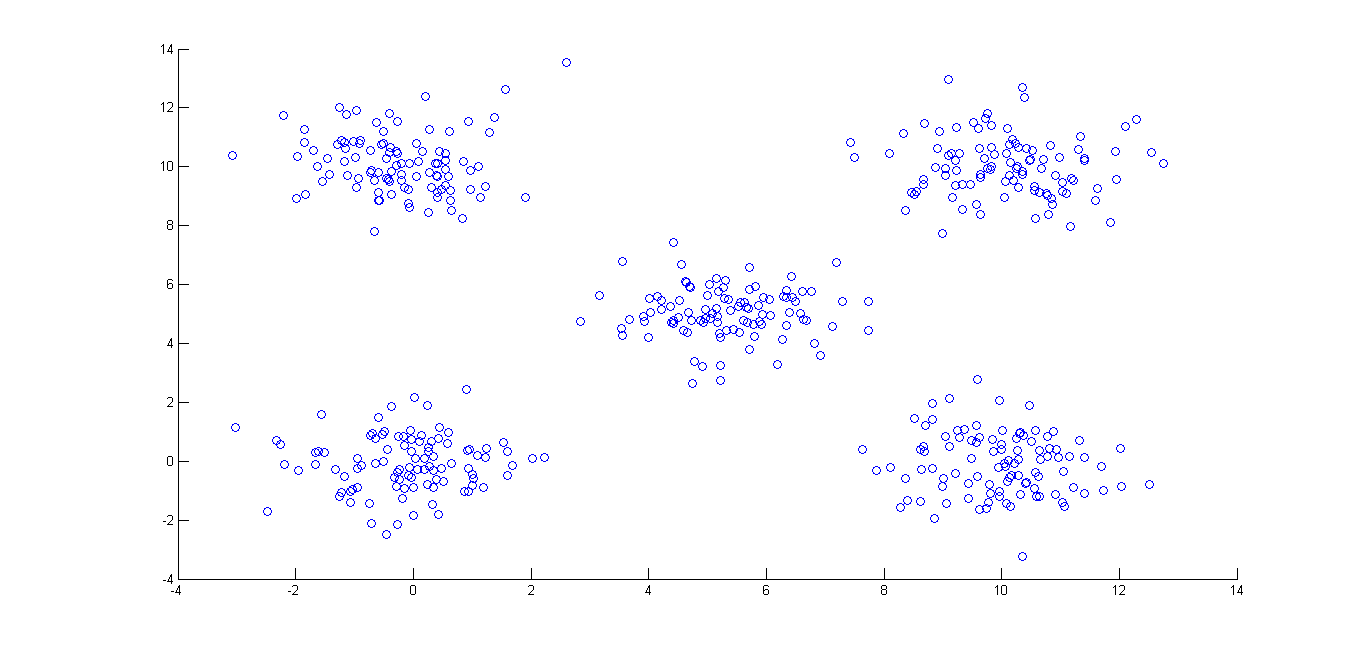然后利用k平均算法进行操作
代码如下：

load('2d-data.mat');
x = r(:,1);
y = r(:,2);
kx = [0.1832 0.5979 9.1695 11.3244 5.6329];
ky = [0.0799 9.6767 8.9553 0.7174 5.3970];
kind = zeros(1,500);%存放某一个点属于那一个类
a = zeros(1,5);%存放某一个点分别与五个点之间的距离
s = zeros(1,500);%存放某一个点到它所属类的距离
b = 0;
ssum = 0;
kindnum = 1;
for i=1:500
for j = 1:5
a(j) = sqrt((kx(j)-x(i))^2+(ky(j)-y(i))^2);
end
b = a(1);
for m = 2:5;
if b>a(m)
b = a(m);
kindnum = m;
else
end
end
s(i) = b;
kind(i) = kindnum;
kindnum = 1;
end

%计算误差和
for i=1:500
ssum = ssum + s(i);
end

kjnum = 0;%属于某一类的数量
kjxsum = 0;
kjysum = 0;
%循环之以求最佳
for forir=1:6

%求所有属于某一类的平均值
for j = 1:5
for m = 1:500
if kind(m)==j
kjnum = kjnum+1;
kjxsum = kjxsum + x(m);
kjysum = kjysum + y(m);
else
end
end
%新的临时中心点
kx(j) = kjxsum/kjnum;
ky(j) = kjysum/kjnum;
kjnum = 0;
kjxsum = 0;
kjysum = 0;
end

for i=1:500
for j = 1:5
a(j) = sqrt((kx(j)-x(i))^2+(ky(j)-y(i))^2);
end
b = a(1);
for m = 2:5;
if b>a(m)
b = a(m);
kindnum = m;
else
end
end
s(i) = b;
kind(i) = kindnum;
kindnum = 1;
end
end

ssum = 0;
%计算误差和
for i=1:500
ssum = ssum + s(i);
end

x1i = 1;
x2i = 1;
x3i = 1;
x4i = 1;
x5i = 1;

for i=1:500
if kind(i)==1
x1(x1i) = x(i);
y1(x1i) = y(i);
x1i = x1i + 1;
else if kind(i) == 2
x2(x2i) = x(i);
y2(x2i) = y(i);
x2i = x2i+1;
else if kind(i) == 3
x3(x3i) = x(i);
y3(x3i) = y(i);
x3i = x3i+1;
else if kind(i) == 4
x4(x4i) = x(i);
y4(x4i) = y(i);
x4i = x4i+1;
else
x5(x5i) = x(i);
y5(x5i) = y(i);
x5i = x5i+1;
end
end
end
end
end

scatter(x1,y1,3)
hold on;
scatter(x2,y2,3)
hold on;
scatter(x3,y3,3)
hold on;
scatter(x4,y4,3)
hold on;
scatter(x5,y5,3)
hold on;
scatter(kx,ky,90,'p')
hold on;
ssumempty

这里，我使用了“半自动”的模式，通过修改循环的次数，直到误差和不再变化（同时也是最小）。结果如下所示，其中的五角星为每一类的平均值点。五个平均值的坐标分别为（-0.1108，-0.0647），（-0.2540，10.1273），（10.0842,10.0162），（9.9627，-0.0961）和（5.3281，5.0506） 。此时的欧式距离和为612.5909。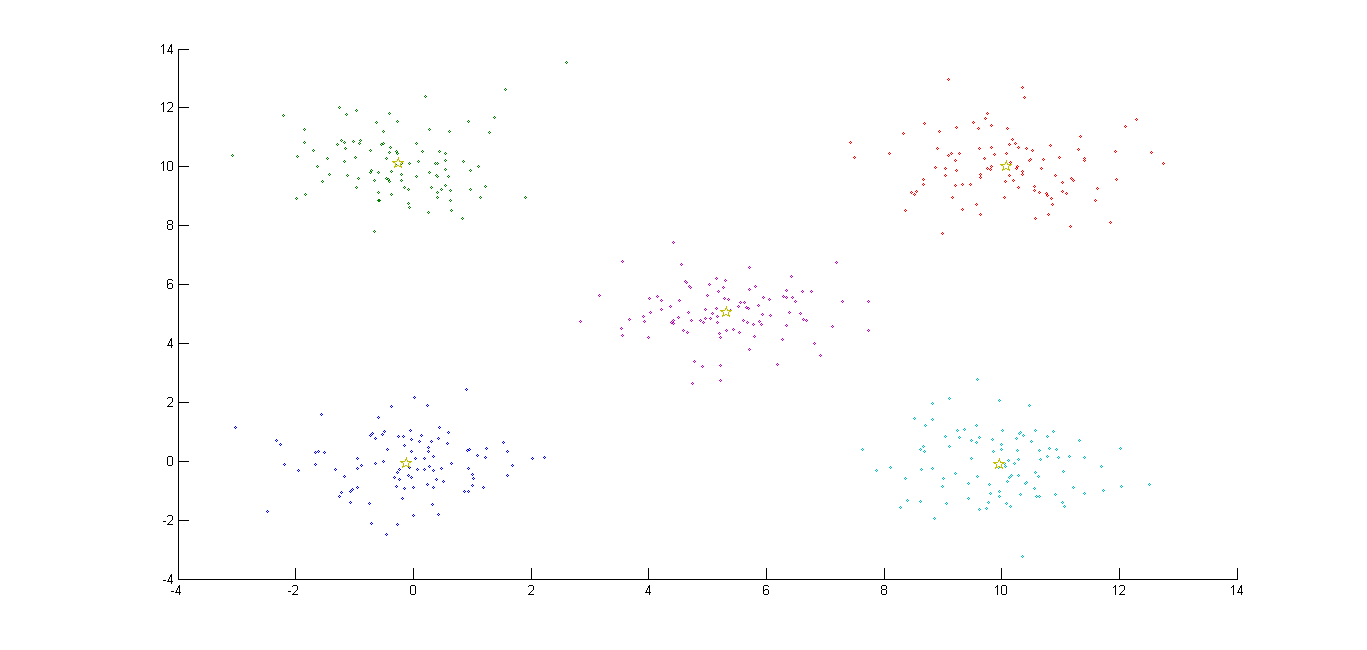三维问题的解决
先将数据的散点图绘制出来观察数据规律
代码如下：
x = r(:,1);
y = r(:,2);
z = r(:,3);
scatter3(x,y,z)

图像如下：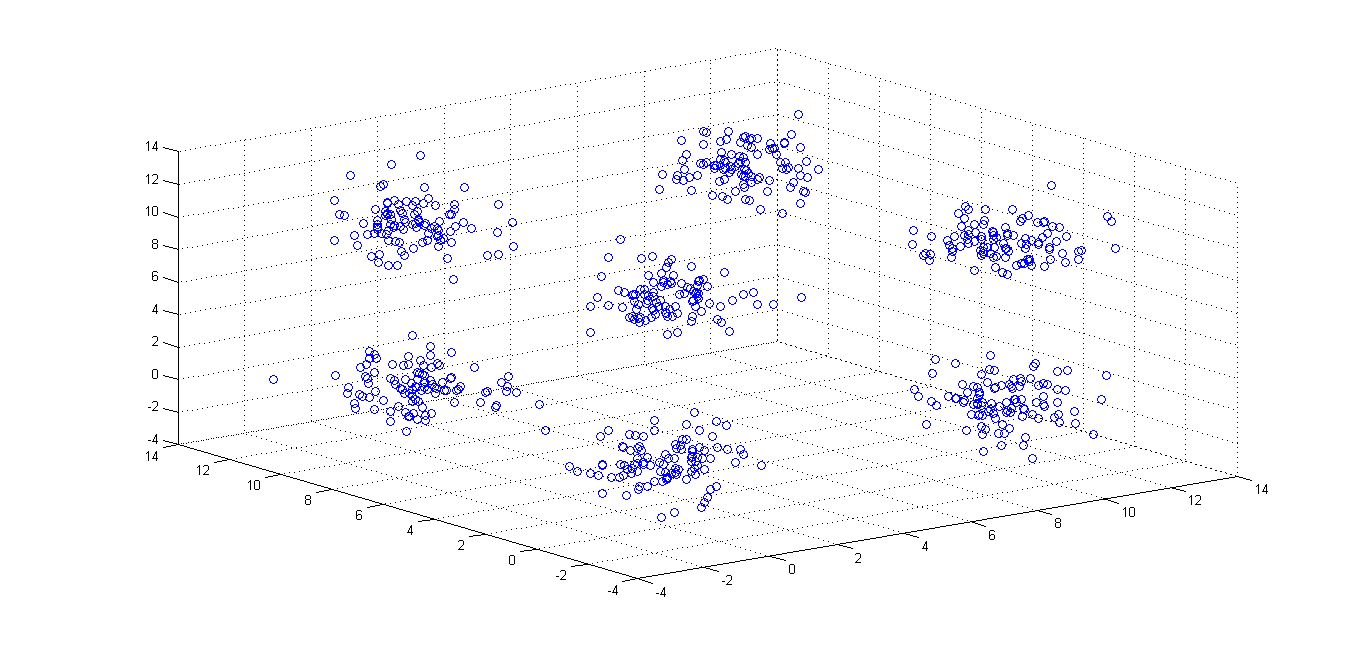用k平均算法进行聚类。
代码如下：

load('3d-data.mat');
x = r(:,1);
y = r(:,2);
z = r(:,3);
kx = [9.7230 1.0262 1.5833 0.4717 -1.6163 10.4582 10.8928];
ky = [9.5779 0.4131 0.9143 9.1354 10.9840 0.6157 0.3589];
kz = [11.5846 0.1446 9.3184 0.7930 9.0882 0.7019 10.9018];
kind = zeros(1,700);%存放某一个点属于那一个类
a = zeros(1,7);%存放某一个点分别与七个点之间的距离
s = zeros(1,700);%存放某一个点到它所属类中心的距离
b = 0;
ssum = 0;%误差和
kindnum = 1;
for i=1:700
for j = 1:7
a(j) = sqrt((kx(j)-x(i))^2+(ky(j)-y(i))^2+(kz(j)-z(i))^2);
end
b = a(1);
for m = 2:7;
if b>a(m)
b = a(m);
kindnum = m;
else
end
end
s(i) = b;
kind(i) = kindnum;
kindnum = 1;
end

%计算误差和
for i=1:700
ssum = ssum + s(i);
end

kjnum = 0;%属于某一类的数量
kjxsum = 0;
kjysum = 0;
kjzsum = 0;
%循环之以求最佳
for forir=1:8

%求所有属于某一类的平均值
for j = 1:7
for m = 1:700
if kind(m)==j
kjnum = kjnum + 1;
kjxsum = kjxsum + x(m);
kjysum = kjysum + y(m);
kjzsum = kjzsum + z(m);
else
end
end
%新的临时中心点
kx(j) = kjxsum/kjnum;
ky(j) = kjysum/kjnum;
kz(j) = kjzsum/kjnum;
kjnum = 0;
kjxsum = 0;
kjysum = 0;
kjzsum = 0;
end

%重新分类
for i=1:700
for j = 1:7
a(j) = sqrt((kx(j)-x(i))^2+(ky(j)-y(i))^2+(kz(j)-z(i))^2);
end
b = a(1);
for m = 2:7;
if b>a(m)
b = a(m);
kindnum = m;
else
end
end
s(i) = b;
kind(i) = kindnum;
kindnum = 1;
end
end

ssum = 0;
%计算误差和
for i=1:500
ssum = ssum + s(i);
end
ssum
%找出所属类以画图
x1i = 1;
x2i = 1;
x3i = 1;
x4i = 1;
x5i = 1;
x6i = 1;
x7i = 1;

for i=1:700
if kind(i)==1
x1(x1i) = x(i);
y1(x1i) = y(i);
z1(x1i) = z(i);
x1i = x1i + 1;
else if kind(i) == 2
x2(x2i) = x(i);
y2(x2i) = y(i);
z2(x2i) = z(i);
x2i = x2i+1;
else if kind(i) == 3
x3(x3i) = x(i);
y3(x3i) = y(i);
z3(x3i) = z(i);
x3i = x3i+1;
else if kind(i) == 4
x4(x4i) = x(i);
y4(x4i) = y(i);
z4(x4i) = z(i);
x4i = x4i+1;
else if kind(i) == 5
x5(x5i) = x(i);
y5(x5i) = y(i);
z5(x5i) = z(i);
x5i = x5i+1;
else if kind(i) == 6
x6(x6i) = x(i);
y6(x6i) = y(i);
z6(x6i) = z(i);
x6i = x6i+1;
else
x7(x7i) = x(i);
y7(x7i) = y(i);
z7(x7i) = z(i);
x7i = x7i+1;
end
end
end
end
end
end
end

scatter3(x1,y1,z1,3)
hold on;
scatter3(x2,y2,z2,3)
hold on;
scatter3(x3,y3,z3,3)
hold on;
scatter3(x4,y4,z4,3)
hold on;
scatter3(x5,y5,z5,3)
hold on;
scatter3(x6,y6,z6,3)
hold on;
scatter3(x7,y7,z7,3)
hold on;
scatter3(kx,ky,kz,90,'p')
hold on;

这里的基本思想和二维时的情景是一样的。结果图像如下所示。七个类中心坐标分别为：（9.9641,10.0875，9.9957），（-0.1625，-0.0271,0.0249），（-0.0105，-0.0366,10.1016），（0.1838,10.0791，-0.0820），（-0.0374,9.9986,10.0079），（10.1295,0.0617,0.0603）和（9.9811，-0.1085,10.0376）。欧氏距离和为790.5695。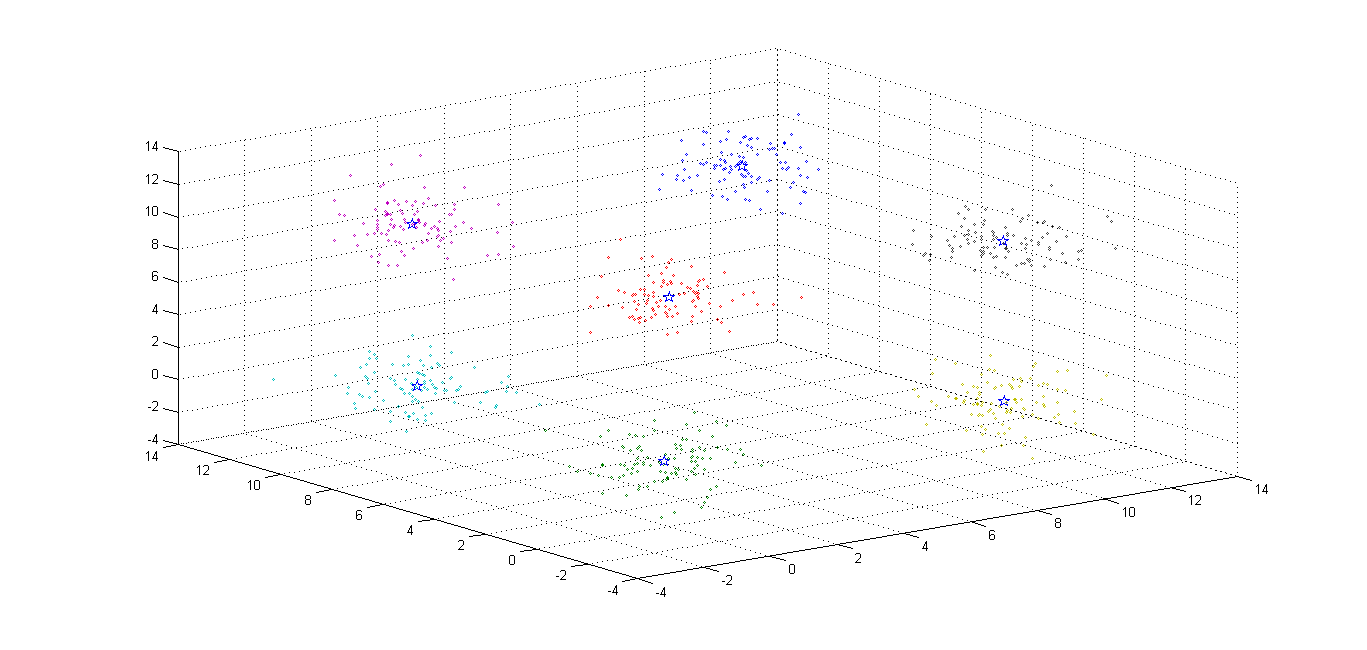收获体会

K平均算法在当时学习数据库系统概念课程的时候最后提到过。但是一直没有亲手来通过数据进行运用。这次小试牛刀感觉还可以。


展开全文机器学习 MATLAB 数据
• 介绍了改进的按距离加权平均法平面数据点规则化的数学模型；讨论了影响方法精度的有关参数。利用两个球体迭加作为理论模型进行了试算，与按距离加权最小二乘法、曲面样条法相比较，本方法的精度较高，平均相对误差&...
• 为了讨论它们的距离关系,建立了概率空间和特征正交空间的最小平均距离问题,并利用模拟退火算法进行求解.进一步利用这个最小距离,获得Burgers方程的一个近似解,而它是由特征正交空间的基线性组合得到的.
• 典型K平均算法中的聚类数k必须是事先给定的确定值,然而实际中很难精确确定,因而无法解决该核算的实际问题。为此，提出距离代价函数作为最佳聚类数的有效性检验函数,建立了相应的数学模型,并据此提出了一种改进的k...
• 1、平均：问题：已知平均分布在圆上的n个点（xi，yi），拟合出圆（即求解出圆心坐标及半径）方法：理论上圆心坐标（a,b）：，圆半径：。
1、平均值法：
问题：已知平均分布在圆上的n个点（xi，yi），拟合出圆（即求解出圆心坐标及半径）
方法：理论上圆心坐标（a,b）：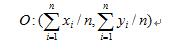，圆半径：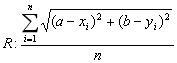。
展开全文• ## 各种距离算法

千次阅读 2017-12-20 20:17:18
1. 欧氏距离，最常见的两点之间或多点之间的距离表示，又称之为欧几里得度量，它定义于欧几里得空间中，如点 x = (x1,...,xn) 和 y = (y1,...,yn) 之间的距离为：   (1)二维平面上两点a(x1,y1)与b(x2,y2)间的...
1. 欧氏距离，最常见的两点之间或多点之间的距离表示法，又称之为欧几里得度量，它定义于欧几里得空间中，如点 x = (x1,...,xn) 和 y = (y1,...,yn) 之间的距离为：(1)二维平面上两点a(x1,y1)与b(x2,y2)间的欧氏距离：(2)三维空间两点a(x1,y1,z1)与b(x2,y2,z2)间的欧氏距离：(3)两个n维向量a(x11,x12,…,x1n)与 b(x21,x22,…,x2n)间的欧氏距离：也可以用表示成向量运算的形式：其上，二维平面上两点欧式距离，代码可以如下编写：

//unixfy：计算欧氏距离doubleeuclideanDistance(constvector<</span>double>&v1, constvector<</span>double>&v2) { assert(v1.size()== v2.size()); doubleret = 0.0; for(vector<</span>double>::size_typei = 0; i != v1.size(); ++i) { ret+= (v1[i] - v2[i]) * (v1[i] - v2[i]); } returnsqrt(ret); }

2. 曼哈顿距离，我们可以定义曼哈顿距离的正式意义为L1-距离或城市区块距离，也就是在欧几里得空间的固定直角坐标系上两点所形成的线段对轴产生的投影的距离总和。例如在平面上，坐标（x1,y1）的点P1与坐标（x2, y2）的点P2的曼哈顿距离为：，要注意的是，曼哈顿距离依赖座标系统的转度，而非系统在座标轴上的平移或映射。

通俗来讲，想象你在曼哈顿要从一个十字路口开车到另外一个十字路口，驾驶距离是两点间的直线距离吗？显然不是，除非你能穿越大楼。而实际驾驶距离就是这个“曼哈顿距离”，此即曼哈顿距离名称的来源，同时，曼哈顿距离也称为城市街区距离(CityBlock distance)。

(1)二维平面两点a(x1,y1)与b(x2,y2)间的曼哈顿距离(2)两个n维向量a(x11,x12,…,x1n)与 b(x21,x22,…,x2n)间的曼哈顿距离3. 切比雪夫距离，若二个向量或二个点p 、and q，其座标分别为及，则两者之间的切比雪夫距离定义如下：，
这也等于以下Lp度量的极值：，因此切比雪夫距离也称为L∞度量。

以数学的观点来看，切比雪夫距离是由一致范数（uniform norm）（或称为上确界范数）所衍生的度量，也是超凸度量（injectivemetric space）的一种。
在平面几何中，若二点p及q的直角坐标系坐标为及，则切比雪夫距离为：。

玩过国际象棋的朋友或许知道，国王走一步能够移动到相邻的8个方格中的任意一个。那么国王从格子(x1,y1)走到格子(x2,y2)最少需要多少步？。你会发现最少步数总是max(| x2-x1 | , | y2-y1 | ) 步 。有一种类似的一种距离度量方法叫切比雪夫距离。

(1)二维平面两点a(x1,y1)与b(x2,y2)间的切比雪夫距离(2)两个n维向量a(x11,x12,…,x1n)与 b(x21,x22,…,x2n)间的切比雪夫距离这个公式的另一种等价形式是4. 闵可夫斯基距离(Minkowski Distance)，闵氏距离不是一种距离，而是一组距离的定义。

(1) 闵氏距离的定义
两个n维变量a(x11,x12,…,x1n)与 b(x21,x22,…,x2n)间的闵可夫斯基距离定义为：其中p是一个变参数。

当p=1时，就是曼哈顿距离

当p=2时，就是欧氏距离

当p→∞时，就是切比雪夫距离

根据变参数的不同，闵氏距离可以表示一类的距离。

5. 标准化欧氏距离 (Standardized Euclidean distance )，标准化欧氏距离是针对简单欧氏距离的缺点而作的一种改进方案。标准欧氏距离的思路：既然数据各维分量的分布不一样，那先将各个分量都“标准化”到均值、方差相等。至于均值和方差标准化到多少，先复习点统计学知识。   假设样本集X的数学期望或均值(mean)为m，标准差(standard deviation，方差开根)为s，那么X的“标准化变量”X*表示为：(X-m）/s，而且标准化变量的数学期望为0，方差为1。 即，样本集的标准化过程(standardization)用公式描述就是：标准化后的值 = ( 标准化前的值 － 分量的均值 ) /分量的标准差　　 经过简单的推导就可以得到两个n维向量a(x11,x12,…,x1n)与 b(x21,x22,…,x2n)间的标准化欧氏距离的公式：如果将方差的倒数看成是一个权重，这个公式可以看成是一种加权欧氏距离(Weighted Euclidean distance)。 6. 马氏距离(Mahalanobis Distance)

（1）马氏距离定义

有M个样本向量X1~Xm，协方差矩阵记为S，均值记为向量μ，则其中样本向量X到u的马氏距离表示为：（协方差矩阵中每个元素是各个矢量元素之间的协方差Cov(X,Y)，Cov(X,Y) =E{ [X-E(X)] [Y-E(Y)]}，其中E为数学期望） 而其中向量Xi与Xj之间的马氏距离定义为：若协方差矩阵是单位矩阵（各个样本向量之间独立同分布）,则公式就成了：也就是欧氏距离了。

若协方差矩阵是对角矩阵，公式变成了标准化欧氏距离。 (2)马氏距离的优缺点：量纲无关，排除变量之间的相关性的干扰。 「微博上的seafood高清版点评道：原来马氏距离是根据协方差矩阵演变，一直被老师误导了，怪不得看Killian在05年NIPS发表的LMNN论文时候老是看到协方差矩阵和半正定，原来是这回事」 7、巴氏距离（Bhattacharyya Distance），在统计中，Bhattacharyya距离测量两个离散或连续概率分布的相似性。它与衡量两个统计样品或种群之间的重叠量的Bhattacharyya系数密切相关。Bhattacharyya距离和Bhattacharyya系数以20世纪30年代曾在印度统计研究所工作的一个统计学家A. Bhattacharya命名。同时，Bhattacharyya系数可以被用来确定两个样本被认为相对接近的，它是用来测量中的类分类的可分离性。

（1）巴氏距离的定义
对于离散概率分布 p和q在同一域 X，它被定义为：其中：是Bhattacharyya系数。
对于连续概率分布，Bhattacharyya系数被定义为：在这两种情况下，巴氏距离并没有服从三角不等式.（值得一提的是，Hellinger距离不服从三角不等式）。
对于多变量的高斯分布，，

和是手段和协方差的分布。
需要注意的是，在这种情况下，第一项中的Bhattacharyya距离与马氏距离有关联。
（2）Bhattacharyya系数
Bhattacharyya系数是两个统计样本之间的重叠量的近似测量，可以被用于确定被考虑的两个样本的相对接近。
计算Bhattacharyya系数涉及集成的基本形式的两个样本的重叠的时间间隔的值的两个样本被分裂成一个选定的分区数，并且在每个分区中的每个样品的成员的数量，在下面的公式中使用考虑样品a 和 b ，n是的分区数，并且，被一个和 b i的日分区中的样本数量的成员。更多介绍请参看：
http://en.wikipedia.org/wiki/Bhattacharyya_coefficient。

8. 汉明距离(Hamming distance)，两个等长字符串s1与s2之间的汉明距离定义为将其中一个变为另外一个所需要作的最小替换次数。例如字符串“1111”与“1001”之间的汉明距离为2。应用：信息编码（为了增强容错性，应使得编码间的最小汉明距离尽可能大）。

或许，你还没明白我再说什么，不急，看下上篇blog中第78题的第3小题整理的一道面试题目，便一目了然了。如下图所示：//动态规划： //f[i,j]表示s[0...i]与t[0...j]的最小编辑距离。f[i,j] = min { f[i-1,j]+1, f[i,j-1]+1, f[i-1,j-1]+(s[i]==t[j]?0:1) }  //分别表示：添加1个，删除1个，替换1个（相同就不用替换）。

与此同时，面试官还可以继续问下去：那么，请问，如何设计一个比较两篇文章相似性的算法？（这个问题的讨论可以看看这里：http://t.cn/zl82CAH，及这里关于simhash算法的介绍：http://www.cnblogs.com/linecong/archive/2010/08/28/simhash.html），接下来，便引出了下文关于夹角余弦的讨论。
（上篇blog中第78题的第3小题给出了多种方法，读者可以参看之。同时，程序员编程艺术系列第二十八章将详细阐述这个问题）

9. 夹角余弦(Cosine) ，几何中夹角余弦可用来衡量两个向量方向的差异，机器学习中借用这一概念来衡量样本向量之间的差异。

(1)在二维空间中向量A(x1,y1)与向量B(x2,y2)的夹角余弦公式：(2) 两个n维样本点a(x11,x12,…,x1n)和b(x21,x22,…,x2n)的夹角余弦类似的，对于两个n维样本点a(x11,x12,…,x1n)和b(x21,x22,…,x2n)，可以使用类似于夹角余弦的概念来衡量它们间的相似程度，即：夹角余弦取值范围为[-1,1]。夹角余弦越大表示两个向量的夹角越小，夹角余弦越小表示两向量的夹角越大。当两个向量的方向重合时夹角余弦取最大值1，当两个向量的方向完全相反夹角余弦取最小值-1。

10. 杰卡德相似系数(Jaccard similarity coefficient)

(1) 杰卡德相似系数
两个集合A和B的交集元素在A，B的并集中所占的比例，称为两个集合的杰卡德相似系数，用符号J(A,B)表示。杰卡德相似系数是衡量两个集合的相似度一种指标。
(2) 杰卡德距离
与杰卡德相似系数相反的概念是杰卡德距离(Jaccard distance)。
杰卡德距离可用如下公式表示：杰卡德距离用两个集合中不同元素占所有元素的比例来衡量两个集合的区分度。
(3) 杰卡德相似系数与杰卡德距离的应用 可将杰卡德相似系数用在衡量样本的相似度上。
举例：样本A与样本B是两个n维向量，而且所有维度的取值都是0或1，例如：A(0111)和B(1011)。我们将样本看成是一个集合，1表示集合包含该元素，0表示集合不包含该元素。
M11 ：样本A与B都是1的维度的个数
M01：样本A是0，样本B是1的维度的个数
M10：样本A是1，样本B是0 的维度的个数
M00：样本A与B都是0的维度的个数
依据上文给的杰卡德相似系数及杰卡德距离的相关定义，样本A与B的杰卡德相似系数J可以表示为：这里M11+M01+M10可理解为A与B的并集的元素个数，而M11是A与B的交集的元素个数。而样本A与B的杰卡德距离表示为J'：11.皮尔逊系数(Pearson Correlation Coefficient)
在具体阐述皮尔逊相关系数之前，有必要解释下什么是相关系数 ( Correlation coefficient )与相关距离(Correlation distance)。
相关系数 ( Correlation coefficient )的定义是：(其中，E为数学期望或均值，D为方差，D开根号为标准差，E{ [X-E(X)] [Y-E(Y)]}称为随机变量X与Y的协方差，记为Cov(X,Y)，即Cov(X,Y) = E{ [X-E(X)] [Y-E(Y)]}，而两个变量之间的协方差和标准差的商则称为随机变量X与Y的相关系数，记为)
相关系数衡量随机变量X与Y相关程度的一种方法，相关系数的取值范围是[-1,1]。相关系数的绝对值越大，则表明X与Y相关度越高。当X与Y线性相关时，相关系数取值为1（正线性相关）或-1（负线性相关）。
具体的，如果有两个变量：X、Y，最终计算出的相关系数的含义可以有如下理解：
当相关系数为0时，X和Y两变量无关系。当X的值增大（减小），Y值增大（减小），两个变量为正相关，相关系数在0.00与1.00之间。当X的值增大（减小），Y值减小（增大），两个变量为负相关，相关系数在-1.00与0.00之间。
相关距离的定义是：OK，接下来，咱们来重点了解下皮尔逊相关系数。
在统计学中，皮尔逊积矩相关系数（英语：Pearson product-moment correlation coefficient，又称作 PPMCC或PCCs, 用r表示）用于度量两个变量X和Y之间的相关（线性相关），其值介于-1与1之间。
通常情况下通过以下取值范围判断变量的相关强度： 相关系数 0.8-1.0极强相关 0.6-0.8强相关 0.4-0.6中等程度相关 0.2-0.4弱相关 0.0-0.2极弱相关或无相关
在自然科学领域中，该系数广泛用于度量两个变量之间的相关程度。它是由卡尔·皮尔逊从弗朗西斯·高尔顿在19世纪80年代提出的一个相似却又稍有不同的想法演变而来的。这个相关系数也称作“皮尔森相关系数r”。
(1)皮尔逊系数的定义：
两个变量之间的皮尔逊相关系数定义为两个变量之间的协方差和标准差的商：以上方程定义了总体相关系数, 一般表示成希腊字母ρ(rho)。基于样本对协方差和方差进行估计，可以得到样本标准差, 一般表示成r：一种等价表达式的是表示成标准分的均值。基于(Xi, Yi)的样本点，样本皮尔逊系数是其中、及，分别是标准分、样本平均值和样本标准差。
或许上面的讲解令你头脑混乱不堪，没关系，我换一种方式讲解，如下：
假设有两个变量X、Y，那么两变量间的皮尔逊相关系数可通过以下公式计算：
公式一：注：勿忘了上面说过，“皮尔逊相关系数定义为两个变量之间的协方差和标准差的商”，其中标准差的计算公式为：公式二：公式三：公式四：以上列出的四个公式等价，其中E是数学期望，cov表示协方差，N表示变量取值的个数。

(2)皮尔逊相关系数的适用范围
当两个变量的标准差都不为零时，相关系数才有定义，皮尔逊相关系数适用于：
两个变量之间是线性关系，都是连续数据。两个变量的总体是正态分布，或接近正态的单峰分布。两个变量的观测值是成对的，每对观测值之间相互独立。

(3)如何理解皮尔逊相关系数

rubyist：皮尔逊相关系数理解有两个角度
其一, 按照高中数学水平来理解, 它很简单, 可以看做将两组数据首先做Z分数处理之后, 然后两组数据的乘积和除以样本数，Z分数一般代表正态分布中, 数据偏离中心点的距离.等于变量减掉平均数再除以标准差.(就是高考的标准分类似的处理)
样本标准差则等于变量减掉平均数的平方和，再除以样本数，最后再开方，也就是说，方差开方即为标准差，样本标准差计算公式为：所以, 根据这个最朴素的理解,我们可以将公式依次精简为:其二, 按照大学的线性数学水平来理解, 它比较复杂一点,可以看做是两组数据的向量夹角的余弦。下面是关于此皮尔逊系数的几何学的解释，先来看一幅图，如下所示：回归直线： y=gx(x) [红色] 和 x=gy(y) [蓝色]

如上图，对于没有中心化的数据, 相关系数与两条可能的回归线y=gx(x) 和 x=gy(y) 夹角的余弦值一致。

对于没有中心化的数据 (也就是说, 数据移动一个样本平均值以使其均值为0), 相关系数也可以被视作由两个随机变量 向量 夹角 的余弦值（见下方）。

举个例子，例如，有5个国家的国民生产总值分别为 10, 20, 30, 50 和 80 亿美元。 假设这5个国家 (顺序相同) 的贫困百分比分别为 11%, 12%, 13%, 15%, and 18% 。 令 x 和 y 分别为包含上述5个数据的向量: x = (1, 2, 3, 5, 8) 和 y = (0.11, 0.12, 0.13, 0.15, 0.18)。
利用通常的方法计算两个向量之间的夹角  (参见 数量积), 未中心化 的相关系数是:我们发现以上的数据特意选定为完全相关: y = 0.10 + 0.01 x。于是，皮尔逊相关系数应该等于1。将数据中心化 (通过E(x) = 3.8移动 x 和通过 E(y) = 0.138 移动 y ) 得到 x = (−2.8, −1.8, −0.8, 1.2, 4.2) 和 y = (−0.028, −0.018, −0.008, 0.012, 0.042), 从中(4)皮尔逊相关的约束条件
从以上解释, 也可以理解皮尔逊相关的约束条件:
1 两个变量间有线性关系2 变量是连续变量3 变量均符合正态分布,且二元分布也符合正态分布4 两变量独立
在实践统计中,一般只输出两个系数,一个是相关系数,也就是计算出来的相关系数大小,在-1到1之间;另一个是独立样本检验系数,用来检验样本一致性。

简单说来，各种“距离”的应用场景简单概括为，空间：欧氏距离，路径：曼哈顿距离，国际象棋国王：切比雪夫距离，以上三种的统一形式:闵可夫斯基距离，加权：标准化欧氏距离，排除量纲和依存：马氏距离，向量差距：夹角余弦，编码差别：汉明距离，集合近似度：杰卡德类似系数与距离，相关：相关系数与相关距离
展开全文• 计算平均值u=(x1+x2+...+xn)/n 计算方差s²=((x1-u)^2 +(x2-u)^2 +...+(xn-u)^2)/n 计算标准差σ=sqrt(s²) //求平均值 double average(double *x, int len) { double sum = 0; for (int i = 0; ...
• 平均欧氏距离是系统聚类中使用最普遍的一个距离指标，它是样品分类的重要依据。为了能使分类者更科学地确定分类阈值，对随机平均欧氏距离的统计性质进行了研究，确定了它的概率分布，给出了它的数字特征，为分类...
• 编写程序计算10个正整数的平均值，找出这10个数中与平均距离（差值的绝对值）最近的三个数，以距离大小对这三个数排序和输出（与平均距离小的先输出）。例如输入{33，44，61，2，36，42，56，81，11，17}，输出...数据结构 c++
• 2、加权平均法问题：针对上一篇平均中存在的缺点：实际中各点不可能均匀分布在圆上，用平均求得的圆心位置偏向数据点密集的一边，求出的半径也可能偏小。解决思想：L：所有相邻两点之间的弧长之和； Li：点...
• 从K近邻算法距离度量谈到KD树、SIFT+BBF算法前言 前两日，在微博上说：“到今天为止，我至少亏欠了3篇文章待写：1、KD树；2、神经网络；3、编程艺术第28章。你看到，blog内的文章与你于别处所见的任何都不同。于是...
• ## 各种距离算法汇总

千次阅读 2019-08-02 11:25:13
1. 欧氏距离，最常见的两点之间或多点之间的距离表示，又称之为欧几里得度量，它定义于欧几里得空间中，如点 x = (x1,...,xn) 和 y = (y1,...,yn) 之间的距离为： (1)二维平面上两点a(x1,y1)与b(x2,y2)间的...
• 1.打开ArcMap软件。 2.按照图片中的步骤操作输入最邻近分析Average Nearest Neighbor。 3.选中要操作的要素文件（点文件）。 4.按照要求选择和点击。 5.按照路径找到结果保存的html文件，双击html文件。......ArcGIS应用
• 距离权重主要依赖于反距离的幂值，幂参数可基于距输出点的距离来控制已知点对内插值的影响。幂参数是一个正实数，默认值为2。（一般0.5到3的值可获得最合理的结果）。 通过定义更高的幂值，可进一步强调最近点...
• 最大最小距离算法也成为小中取大距离算法。这种方法首先根据确定的距离阈值寻找聚类中心，然后根据最近邻规则把模式样本划分到各聚类中心对应的类别中。 问题的提出 已知N个待分类的模式样本{X1,X2,…,Xn}，...
• 欧氏距离、马氏距离、闵式距离、曼哈顿距离、切比雪夫距离、卡方距离、二值变量距离、Jaccard距离、余弦相似度、汉明距离、皮尔森相关系数、最远（近）距离、重心距离、ward方法（离差平方和距离）、组间平均链锁...层次聚类...